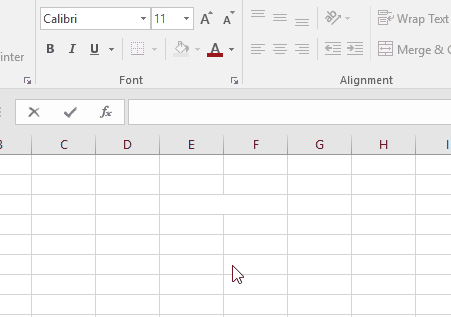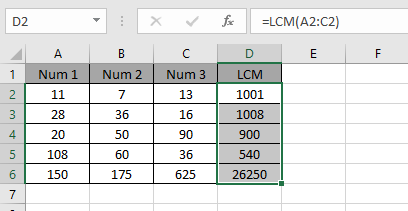# How to use the LCM Function in Excel

LCM is Lowest Common Multiple. LCM of two or more integers is the lowest number which can be divided by every number in the argument. If the numbers input to the formula is prime numbers, just multiply all the input numbers to get the LCM.

Let’s take an example to see how LCM function works.
Let’s first take two number 45 & 54. The factors for the numbers are:

45 : 1 x 9 x 5
54 : 1 x 9 x 3 x 2

The common divisors from the above 2 factors are:

LCM = 1 x 5 x 3 x 2 x 9 = 270

Multiply them to get the LCM of 45 & 54. LCM is 270 i.e. 270 is the lowest number which is common multiple for 45 & 54.The LCM function returns a number representing the LCM of the input numbers. It takes numbers as an argument and returns the LCM for the numbers using the formula.
Syntax:

=LCM (number1, [number2])

Arguments to the function can be given an array on directly supplied integers.
Let’s understand the LCM function via using it on some numbers to get results.To get the LCM for the number shown in the above snapshot.
Use the formula:

=LCM(A2:C2)

Numbers provided in as arrayAs you can see the LCM for prime numbers is just multiple of input prime numbersAs you can see the Lowest Common Multiple or LCM of the numbers is calculated using the formula.

Hope you understood how to use the LCM function in Excel. Explore more articles on Excel mathematical functions here. Please feel free to state your query or feedback for the above article.

Related Article:

How to use the GCD Function in Excel

Popular Articles:

50 Excel Shortcuts to Increase Your Productivity

How to use the VLOOKUP Function in Excel

How to use the COUNTIF in Excel 2016

How to use the SUMIF Function in Excel

Terms and Conditions of use

The applications/code on this site are distributed as is and without warranties or liability. In no event shall the owner of the copyrights, or the authors of the applications/code be liable for any loss of profit, any problems or any damage resulting from the use or evaluation of the applications/code.